#Function Repository Resource:

# MiniMaxApproximation

Find a mini-max approximation of a function

Contributed by: Wolfram Research
 ResourceFunction["MiniMaxApproximation"][expr,{x,{x0,x1},m,n}] finds the rational polynomial function of x, with numerator order m and denominator order n, that gives a mini-max approximation to expr on the interval x0 to x1. ResourceFunction["MiniMaxApproximation"][expr,approx,{x,{x0,x1},m,n}] finds the mini-max approximation to expr, starting the iterative algorithm with approx.

## Details and Options

ResourceFunction["MiniMaxApproximation"] minimizes the maximum value of the relative error between the approximation and expr.
ResourceFunction["MiniMaxApproximation"] returns {abscissa,{approximation,maxerror}}, where abscissa is a list of the abscissas where the relative error is a local maximum, approximation is the rational approximant and maxerror is the global maximum of the relative error.
When an approximation is given as the second argument of ResourceFunction["MiniMaxApproximation"], it must have the same form as the result returned by ResourceFunction["MiniMaxApproximation"].
The following options can be given:
 "Bias" 0 bias in the automatic choice of interpolation points "Brake" {5,5} braking to apply on the iterative algorithm "Derivatives" Automatic function to use for derivatives MaxIterations 20 maximum number of iterations to use "PlotFlag" False whether to plot relative error "PrintFlag" False whether to print status information WorkingPrecision MachinePrecision precision to use in internal computations
The option "Bias" is used to control the initial rational approximation in the same way it is used in RationalInterpolation.

## Examples

### Basic Examples

A list containing the points where the maximum error occurs and the desired interpolation, along with the value of the error:

 In:=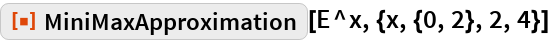Out=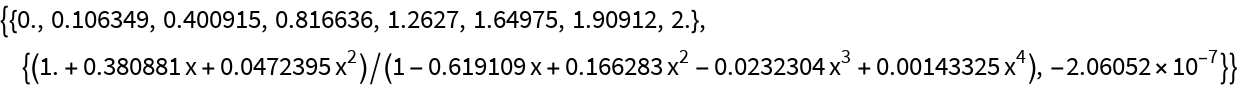The rational approximation:

 In:=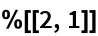Out=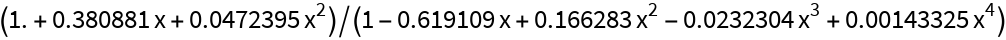The relative error in the approximation over the interval:

 In:=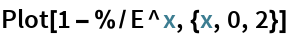Out=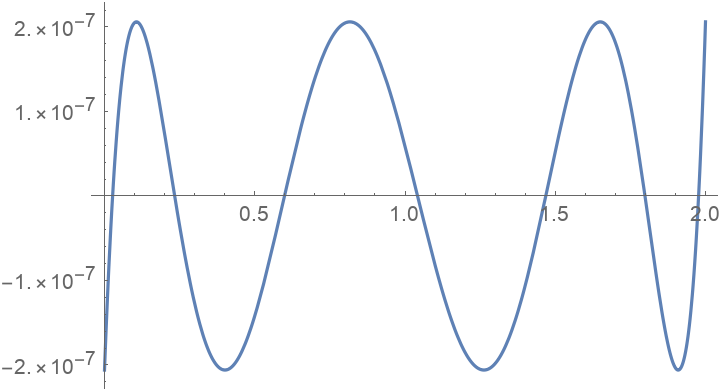### Options

#### Brake

The convergence process does not finish within a small number of iterations without braking:

 In:=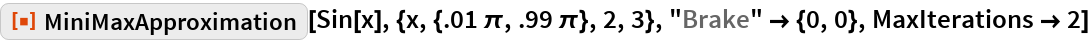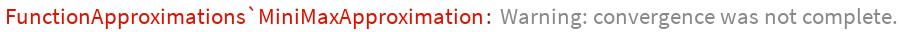Out=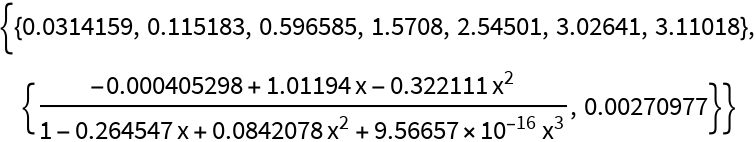With the default brake, the conversion succeeds:

 In:=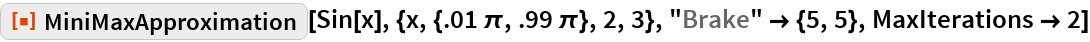Out=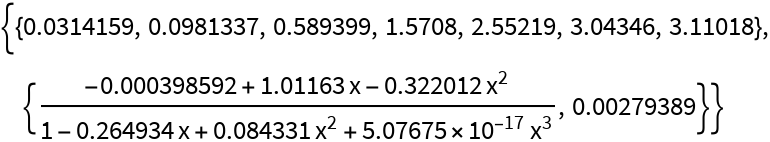#### Derivatives

Supply a function that gives a list of derivatives of the function to be approximated, evaluated at numeric points:

 In:=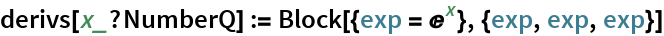In:=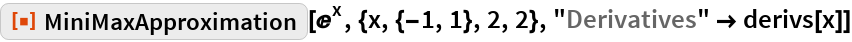Out=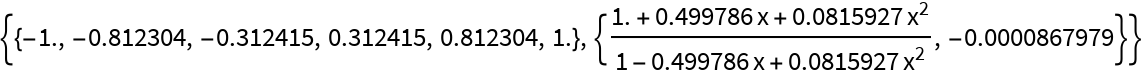### Possible Issues

Because MiniMaxApproximation tries to minimize the maximum of the relative error, it is not possible to find a minimax approximation to a function that has a zero in the interval in question:

 In:=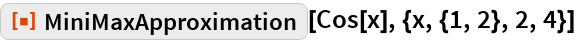Out=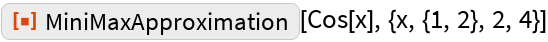Divide out the zero and then multiply back into the rational function:

 In:=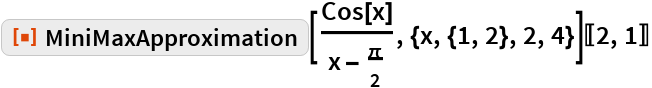Out=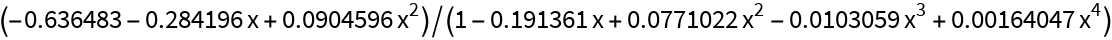In:=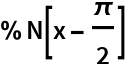Out=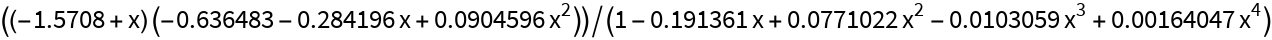The relative error:

 In:=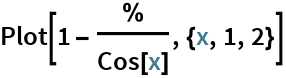Out=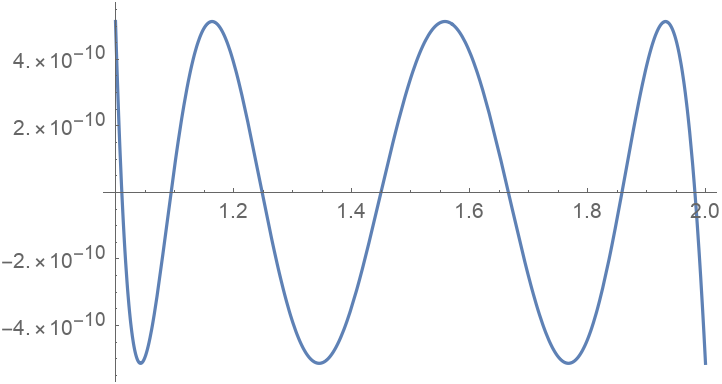## Requirements

Wolfram Language 11.3 (March 2018) or above